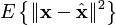# Linear least squares

#### http://en.wikipedia.org/wiki/Linear_least_squares

Limitations
The least squares approach relies on the calculation of the pseudo inverse. The pseudo inverse is guaranteed to exist for any full-rank matrix A. However, in some cases the matrix ATA is ill-conditioned; this occurs when the measurements are only marginally related to the estimated parameters. In these cases, the least squares estimate amplifies the measurement noise, and may be grossly inaccurate. This may occur even when the pseudo inverse itself can be accurately calculated numerically. Various regularization techniques can be applied in such cases, the most common of which is called Tikhonov regularization. If further information about the parameters is known, for example, a range of possible values of x, then minimax techniques can also be used to increase the stability of the solution.

Another drawback of the least squares estimator is the fact that it seeks to minimize the norm of the measurement error, Axb. In many cases, one is truly interested in obtaining small error in the parameter x, e.g., a small value of. However, since x is unknown, this quantity cannot be directly minimized. If a prior probability on x is known, then a Bayes estimator can be used to minimize the mean squared error,. The least squares method is often applied when no prior is known. Surprisingly, however, better estimators can be constructed, an effect known as Stein’s phenomenon. For example, if the measurement error is Gaussian, several estimators are known which dominate, or outperform, the least squares technique; the most common of these is the James-Stein estimator.